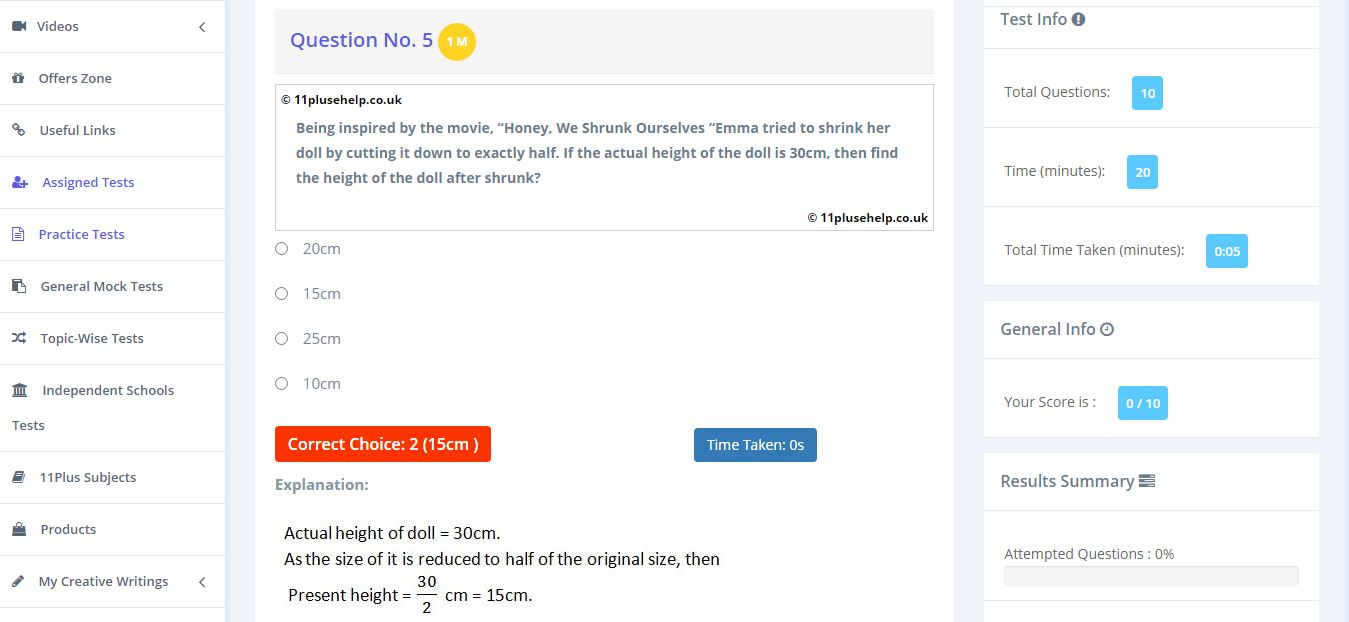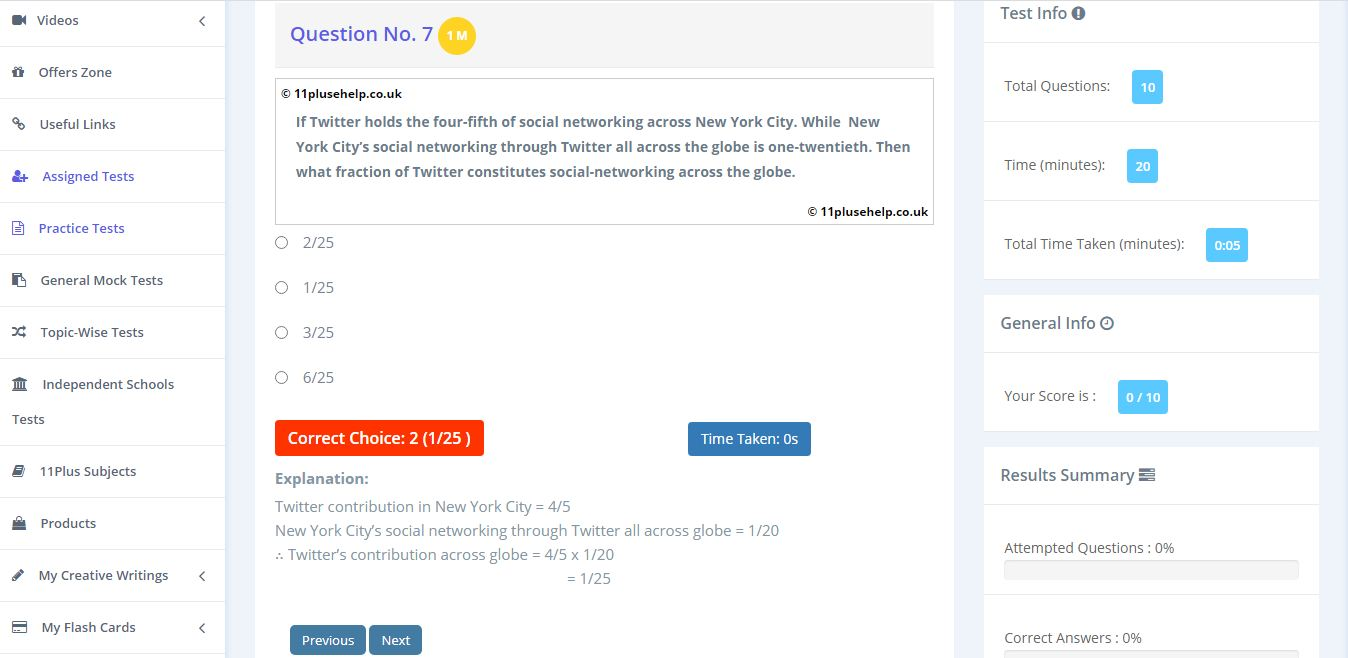# Free 11 Plus Fraction Practice Papers

Free 11 Plus Fraction Practice Papers

Yes! You have read it correctly. There are Free 11 Plus Fraction Practice Papers available in 11PluseHelp.co.uk.

The test papers cover different question types, which will help every child clear the entrance exams like CEM, CSSE, GL Assessment, Independent Schools conducted by different grammar schools, Independent schools and other private schools. To meet the entrance exams’ expectation, we have prepared practice test papers on different levels like Easy, Medium and High. By practising the exam papers on these different levels, children can identify their strengths and weaknesses in 11 Plus Maths papers. 11PluseHelp.co.uk has many Eleven Plus Exam practice tests which help children to focus and improve their skills in all subjects.

# Free 11 Plus Fraction Practice Papers

Fraction

Fractions are considered as representing a part of a whole quantity. Fractions are usually represented in the form of a/b where a, b are 0. The terminologies of fractions are generally used while dividing a thing into smaller parts, like sharing a pizza among friends etc.The pizza shown in the above picture has been divided into eight pieces. When the pizza is considered as a whole, then each part is given by 1/8, which is a fraction.A fraction consists of 2 parts they are Numerator and Denominator.Types of fractions:

Generally, fractions can be divided into three types they are

• Proper Fractions
• Improper fractions
• Mixed Fractions

The fractions in which denominator is greater than the numerator then such fractions are considered as the Proper Fractions. Examples are ½, ¼, ¾, etc.

The fractions in which denominator is greater than the numerator then such fractions are considered as the Improper Fractions. Examples are 11/4, 5/2, 10/7 etc.

The other form of representing an improper fraction is in terms of Mixed Fractions. It is a combination of a whole number and a proper fraction. Examples are 3 ½, 5 ¼, 7 ¾, etc.

Some important facts about fractions are

• The fractions with the same denominator are said to be Like Fractions else they are said as, Unlike Fractions.
• A fraction in which numerator and denominator are equal, then its value will be equal to One. Eg 2/2 = 1.
• A fraction in which the numerator = 0, then the value of the fraction will be Zero. Eg 0/100 = 0.
• A fraction in which denominator = 0, then the fraction is said to be undefined.

Example: Jason brings a cake and cuts it into 6 equal parts and distributes among his friend, what fraction does each friend get?

Sol: No. of pieces = 6, then each one will be getting 1/6 part of the cake.

Arithmetic operations on fractions

In order to perform addition or subtraction, we need to check if the fractions have the same denominator or not. We will know about them in detail through some interesting and easy examples.

Consider two fractions, a/b and c/b, then their sum will be (a + c)/b, similarly in subtraction also, it will be (a-c)/b. Here denominators of both the fractions are equal.

Now Consider two fractions, a/b and c/d then, to find their sum or difference we must make their denominators equal. For this we must find the LCM of the denominators and based on it we must make the fractions like or we follow another method, in which the fractions are represented as (ad/bd), (bc/bd) and perform the addition or subtraction.

Sol: First convert them into like fractions. 1/2 can be written as (1×3) / (2×3) = 3/6, similarly, 2/3 can be written as 2/6. Now we can add both the fractions,

3/6 + 2/6 = 5/6

For multiplication it is very easy, just multiply the numerators and denominators separately. For division just reciprocate the 2nd fraction and multiply.

Multiplication of a / b & c / d = (a x b) / (c x d)

Division of a / b & c / d = (a x d) / (b x c)

Example: Multiply 1/4 and 2/3?

Sol: It is very easy just to multiply numerators and denominators separately.

1/4 x 2/3 = (1 x 2) / (4 x 3) = 2 / 12.

11 plus fractions topic is very important as it will be used in the calculation of percentages and decimals and many other 11 plus maths practice tests. You can access 11 plus maths practice tests on different topics here:

https://www.11plusehelp.co.uk/11-plus-maths-practice-tests

Try out these examples without sneak peeking at the answers:

Example:1Example:2You can access 11 Plus Online Maths Exam Papers on different topics here:

https://www.11plusehelp.co.uk/11-plus-maths-practice-tests

There are many 11 Plus Practice papers for entrance exams, Independent school papers and more for the 11 tests. Ranging from 10 to 25 minutes, our multiple-choice and fill-up question types are ideal for Maths revision. These are similar to Medway test practice papers and Bond Online 11 Plus Practice Papers.

Free 11 Plus Money and Cost Practice Papers:

Free 11 Plus Money and Cost Practice Papers

Free 11 Plus Probability Practice Papers:

https://www.11plusehelp.co.uk/blog/2021/04/06/free-11-plus-probability-practice-papers/

Free 11 Plus Place Value and Rounding Practice Papers:

Free 11 Plus Place Value and Rounding Practice Papers

### FREE Registration:

Registration is FREE. You can access Free tests, Resources and videos after registration. You can also view all the available Maths, English, Verbal, Non-verbal Reasoning and Independent School Tests, Flashcards, videos etc.

Just follow these simple steps of registration (it’s free).

2. Fill in your details and click ‘Submit.’

The same is shown below.Free 11 Plus Maths Topic – Wise Practice Papers:

https://www.11plusehelp.co.uk/blog/2021/03/15/free-11-plus-maths-topic-wise-practice-papers/

Free 11 Plus English Topic – Wise Practice Papers:

https://www.11plusehelp.co.uk/blog/2021/03/15/free-11-plus-english-topic-wise-practice-papers/

Free 11 Plus Verbal Reasoning Topic – Wise Practice Papers:

https://www.11plusehelp.co.uk/blog/2021/03/15/free-11-plus-verbal-reasoning-topic-wise-practice-papers/

Free 11 Plus Non-Verbal Reasoning Topic – Wise Practice Papers:

https://www.11plusehelp.co.uk/blog/2021/03/15/free-11-plus-non-verbal-reasoning-topic-wise-practice-papers/

You can access free 11 Plus practice papers from the below link:

https://www.11plusehelp.co.uk/11-plus-tests/free

11PluseHelp.co.uk is a one-stop-shop for 11 Plus Preparation, including 11 Plus Independent schools preparation.

You can have unlimited online practice on 11 Plus subjects – Maths, English, Verbal Reasoning and Non-Verbal Reasoning.

We focus on fundamentals, logic, basics and cover most of the syllabus for all 11 Plus exam patterns in the UK and Northern Ireland. For example, We cover Maths topic wise questions and fundamentals and how to apply them. We have various tests, time-based, short, long and various types, including standard mocks.  All these tests lead to perfect practice, and the child should be fully prepared to face any 11 Plus Grammar School tests or Independent Schools tests. We provide the free 11 Plus Practice Papers, which are helpful for Bond 11 Plus,11 exam Birmingham and Bucks 11 Plus. 11 Plus Books, Bond 11 Plus books,cem 11 Plus Books and GL 11 Plus Books are available 11 Plus Preparation.

Free 11 Plus Area and Perimeter Practice Papers:

https://www.11plusehelp.co.uk/blog/2021/04/05/free-11-plus-area-and-perimeter-practice-papers/

Free 11 Plus Bidmas Practice Papers:

https://www.11plusehelp.co.uk/blog/2021/04/06/free-11-plus-bidmas-practice-papers/

Practice Mode – Without any time limit for all children ( Year 1, Year 2, Year 3 & Year 4  Year 5 or Year 6 children).

You can access 11 Plus FREE Papers by visiting the below link:
https://www.11plusehelp.co.uk/11-plus-free-online-papers

You can access 11 Plus FREE Sample Papers by visiting the below link:
https://www.11plusehelp.co.uk/11-plus-sample-papers

To access our online virtual mocks visit the link

https://11plus-mockexams.co.uk/

11 Plus complete solution features:

11 Plus Complete Solution……………

Practice and Perseverance Over Genius and Talent

Thanks,

11Plusehelp.co.uk